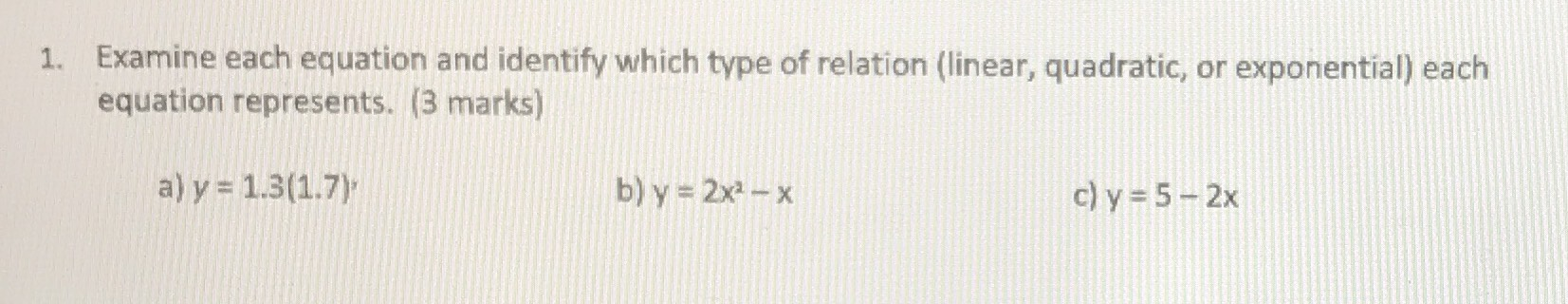### ¿Todavía tienes preguntas de matemáticas?

Pregunte a nuestros tutores expertos
Algebra
Pregunta1. Examine each equation and identify which type of relation (linear, quadratic, or exponential) each equation represents. ( $$3$$ marks)

a) $$y = 1.3 ( 1.7 )$$ b) $$y = 2 x ^ { 2 } - x$$ c) $$y = 5 - 2 x$$